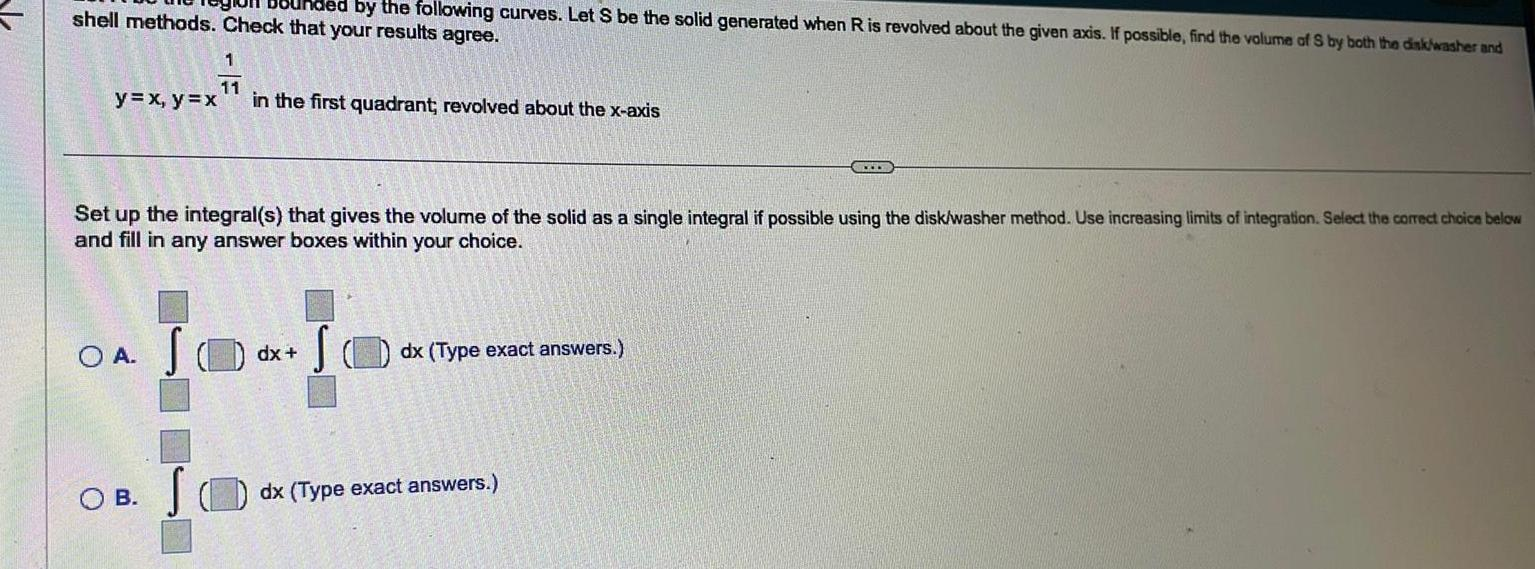Question:

# ided by the following curves Let S be the solid generated

Last updated: 11/19/2023ided by the following curves Let S be the solid generated when R is revolved about the given axis If possible find the volume of S by both the disk washer and shell methods Check that your results agree y x y x OA S 1 11 in the first quadrant revolved about the x axis Set up the integral s that gives the volume of the solid as a single integral if possible using the disk washer method Use increasing limits of integration Select the correct choice below and fill in any answer boxes within your choice OB dx S dx Type exact answers www dx Type exact answers